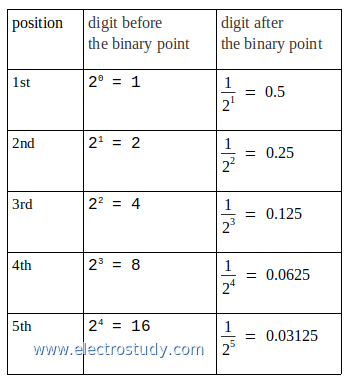# Binary fractions to decimal numbers

This post is explain how to convert binary fractions to decimal numbers. The picture below is show binary fraction to decimal, form 1st position to 5th position of the digit, before and after the binary point.Binary fractions to decimal
Example 1:
Convert binary number 0.1101 to decimal number

Completion:
0.101 (2) = ... (10)
1st digit after binary point: 1 → 1 × (1/ 21) = 1 × 0.5 = 0.5
2nd digit after binary point: 0 → 0 × (1/ 22) = 0 × 0.25 = 0
3rd digit after binary point: 1 → 1 × (1/ 23) = 1 × 0.125 = 0.125
sum all → 0.5 + 0 + 0.125 = 0.625

0.101 (2) = 0.625 (10)

Example 2:
Convert binary number 1110.11011 to decimal number

Completion:
1110.11011 (2) = ... (10)

before binary point
1st digit: 0 → 1 × 20 = 0 × 1 = 0
2nd digit: 1 → 1 × 21 = 1 × 2 = 2
3rd digit: 1 → 1 × 22 = 1 × 4 = 4
4th digit: 1 → 1 × 23 = 1 × 8 = 8
sum all → 0 + 2 + 4 + 8 = 14

after binary point
1st digit: 1 → 1 × (1/ 21) = 1 × 0.5 = 0.5
2nd digit: 1 → 1 × (1/ 22) = 1 × 0.25 = 0.25
3rd digit: 0 → 0 × (1/ 23) = 0 × 0.125 = 0
4th digit: 1 → 1 × (1/ 24) = 1 × 0.0625 = 0.0625
5th digit: 1 → 1 × (1/ 25) = 1 × 0.03125 = 0.03125
sum all → 0.5 + 0.25 + 0 + 0.0625 + 0.03125 = 0.84375

1110.11011 (2) = 14.84375 (10)
Next→
←Prev